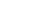Thank you!
Our representative will contact you shortly
Error occurred while submitting data. Please try again after some time.

## Fill in the details below

We will call you back as soon as possibleKnowledge is all about sharing and innovation

# Features & Benefits of EMI calculator in the home loan application processEquated Monthly Installment (EMI) is the amount payable every month to the financial institution until the loan amount is fully paid off. The sum of principal amount and interest is divided by the tenure, i.e., number of months, in which the loan has to be repaid. The interest component of the EMI would gradually reduce with each payment. The exact percentage allocated towards payment of the principal depends on the interest rate. Even though your monthly EMI payment won’t change, the proportion of principal and interest components will change with time. With each successive payment, you’ll pay more towards the principal and less in interest.

Taking a home loan requires good amount of planning, as you are borrowing a hefty sum which needs to be repaid. Therefore, using a home loan EMI calculator can help you plan well.

#### Enter the following information in the EMI Calculator:

Principal loan amount you wish to avail (rupees)

Loan term (months or years)

Rate of interest (percentage)

EMI in advance OR EMI in arrears (for car loan only)

Use the slider to adjust the values in the EMI calculator form. If you need to enter more precise values, you can type the values directly in the relevant boxes provided above. As soon as the values are changed using the slider (or hit the ‘tab’ key after entering the values directly in the input fields), EMI calculator will re-calculate your monthly payment (EMI) amount.

#### Formula for home loan calculator

EMI = [P x R x (1+R)^N]/[(1+R)^N-1],

In this formula, the letters stand for:

EMI is the equated monthly instalment

P is the principal or the amount that is borrowed as a loan

R is the rate of interest that is levied on the loan amount (the interest rate should be a monthly rate)

N is the tenure of repayment of the loan or the number of monthly instalments that you will pay (tenure should be in months)

It is not easy to calculate EMI applicable on your home loan using Excel or by hand, as it is a complicated one. Hence, it is best to use a home loan EMI calculator for the same. Using a housing loan calculator you can calculate the EMI within seconds and save yourself from time-consuming tedious calculations.

#### How it works

Home loan EMI calculator computes the EMI according to the details entered by you in the calculator. The EMI calculator for home loans works on the following inputs:

Loan Amount: This is the sum amount that you want to borrow for purchasing your house. This amount is mostly depend upon the cost of the property as well as your repayment capacity. Bank or financial institution can only provide 80% of the property value as loan. This is the principal amount of the loan and the rate of interest is applied to it. The final amount (post-levy of the interest rate) is then divided equally by the tenure of the loan. You can enter the amount you wish to borrow into the EMI calculator. You can enter the EMI or monthly instalment keeping in mind that it should not be more than 40% of your monthly income.

Interest Rate: It is the rate at which interest is levied on the amount lent. It differs from one financial institution to another, so it is best to check the interest rates before applying for a home loan. You can opt for a fixed rate of interest or floating rate of interest. In fixed Interest rate, the interest rate remains unchanged for the entire loan tenure and all loan EMIs are equal. In the case of floating interest rate, the rates will fluctuate with any change in the base rate and hence the EMIs vary.

Tenure: It is the time allotted to or chosen for repayment of the loan. Most of the loans come with flexible loan tenures. Home loans are have a longer repayment tenure at times even up to 30 years. The longer the tenure you choose the more interest you will have to pay.

#### The importance of calculating EMI

EMI calculator is all about planning. It is always helpful to know what your EMI would be before you have applied for a loan. This will help you to budget your monthly expenses so that your loan will not create a huge impact on your living style.

With every instalment, a part of the principal and a part of the interest is repaid. During the initial phase of the loan tenure, a larger portion of the EMI is allocated towards repayment of interest. Practically after half the tenure has passed, a larger fraction of the EMI is accounted towards principal.

#### Why does the EMI change over the tenure?

In case there is any change in your loan amount, rate of interest or tenure, your EMI might change. Some of the common reasons are:

If you prepays your loan then your principal amount will fall and therefore the interest calculated on the balance amount will change too. This will bring the EMI down.

If your loan is based on flexible rate of interest then the EMI will be adjusted to account for the change in interest rate.

If you ask for a longer loan tenure, then your EMI will be lower.

In order to avoid poor credit, it is advisable to take help of EMI calculator and understand on what will be your left over disposable income ultimately after paying the EMI.